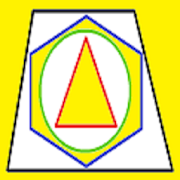## Area, Perimeter, Circumference - Lumos Educational App Store4.21
Price -fREE
\$0

#### DESCRIPTION:

A simple way to find the perimeter, circumference and area for any shape. AREA :square millimeter (mm^2); square centimeter (cm^2); square meter (m^2); square kilometer (km^2); square foot (ft^2); square inch (in^2) LENGTH :millimeter (mm); centimeter (cm); meter (m); kilometer (km); foot (ft); inch(in)

#### OVERVIEW:

Area, Perimeter, Circumference is a free educational mobile app By .It helps students in grades HS practice the following standards HSG.GMD.A.1.

This page not only allows students and teachers download Area, Perimeter, Circumference but also find engaging Sample Questions, Videos, Pins, Worksheets, Books related to the following topics.

1. HSG.GMD.A.1 : Give an informal argument for the formulas for the circumference of a circle area of a circle volume of a cylinder pyramid and cone. Use dissection arguments, Cavalieris principle, and informal limit arguments..

HS

#### STANDARDS:

HSG.GMD.A.1

Developer:

Software Version: 1

Category:

### RELATED APPSEdSearch WebSearch